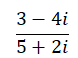# TExMaT Master Mathematics Teacher 8-12 (089): Practice & Study Guide Final Exam

Free Practice Test Instructions:

Choose your answer to the question and click 'Continue' to see how you did. Then click 'Next Question' to answer the next question. When you have completed the free practice test, click 'View Results' to see your results. Good luck!

#### Question 1 1. Solve the following linear inequality.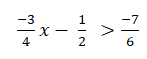#### Question 3 3. Multiply and simplify the following expression.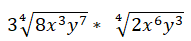#### Question 6 6. Solve the following expression for x.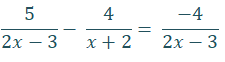#### Question 9 9. Solve the following trig equation for all values of x between 0 and 2π.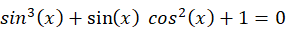#### Question 10 10. Determine where the following function is discontinuous.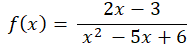#### Question 11 11. Use the given formula to determine the value of x.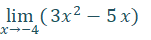#### Question 12 12. Solve for x: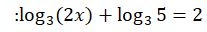#### Question 13 13. Divide the following complex numbers.